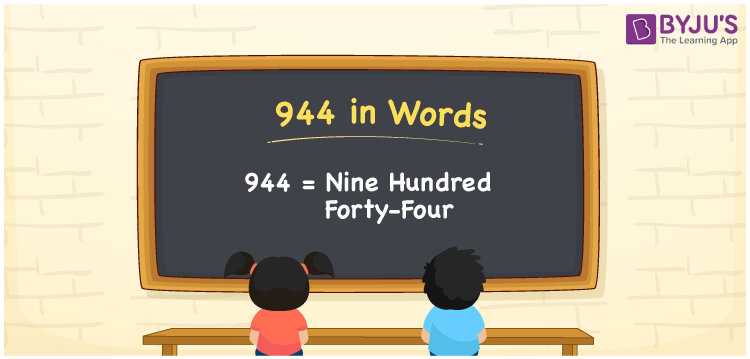# 944 in Words

We can write 944 in words as Nine hundred forty-four. We know that the number name is used to express, spell or convey the number to others. That means the number name of 9 is nine, which is used to spell the number 9. Similarly, the number name of 944 is Nine hundred forty-four. However, it is possible to write the number names for single and double-digit numbers. When it comes to three or more digit numbers, we must use the place value chart to derive their word forms. In this article, you can learn how to derive the word form of 944 using a place value chart in detail.

 944 in words Nine hundred forty-four Nine hundred forty-four in Numbers 944

## 944 in English Words

We generally write numbers in words using the English alphabet. Thus, we can spell 944 in English words as “Nine hundred forty-four”.## How to Write 944 in Words?

In this section, you will learn how to convert 944 into words. Let’s make a three-column chart such that the place value for all three digits of 944 can be assigned. This can be done as given below.

 Hundreds Tens Ones 9 4 4

Here, we can observe that, ones = 4, tens = 4, hundreds = 9

We can write the expanded form as:

9 × Hundred + 4 × Ten + 4 × One

= 9 × 100 + 4 × 10 + 4 × 1

= 900 + 40 + 4

= Nine hundred + Forty + Four

= Nine hundred forty-four

Therefore, 944 in words = Nine hundred forty-four

944 is a natural number that precedes 945 and succeeds 943.

944 in words – Nine hundred forty-four

Is 944 an odd number? – No

Is 944 an even number? – Yes

Is 944 a prime number? – No

Is 944 a composite number? – Yes

Is 944 a perfect square number? – No

Is 944 a perfect cube number? – No

## Frequently Asked Questions on 944 in Words

### What is the spelling of 944?

The spelling of 944 in English words is “Nine hundred forty-four”.

### How do you write Rs. 944 in words on a cheque?

On a cheque, we can write Rs. 944 in words as “Nine hundred forty-four rupees only”.

### Write the value of 944 + 630 in words.

944 + 630 = 1574 Therefore, the value of 944 + 630, i.e. 1574 in words is One thousand five hundred seventy-four.